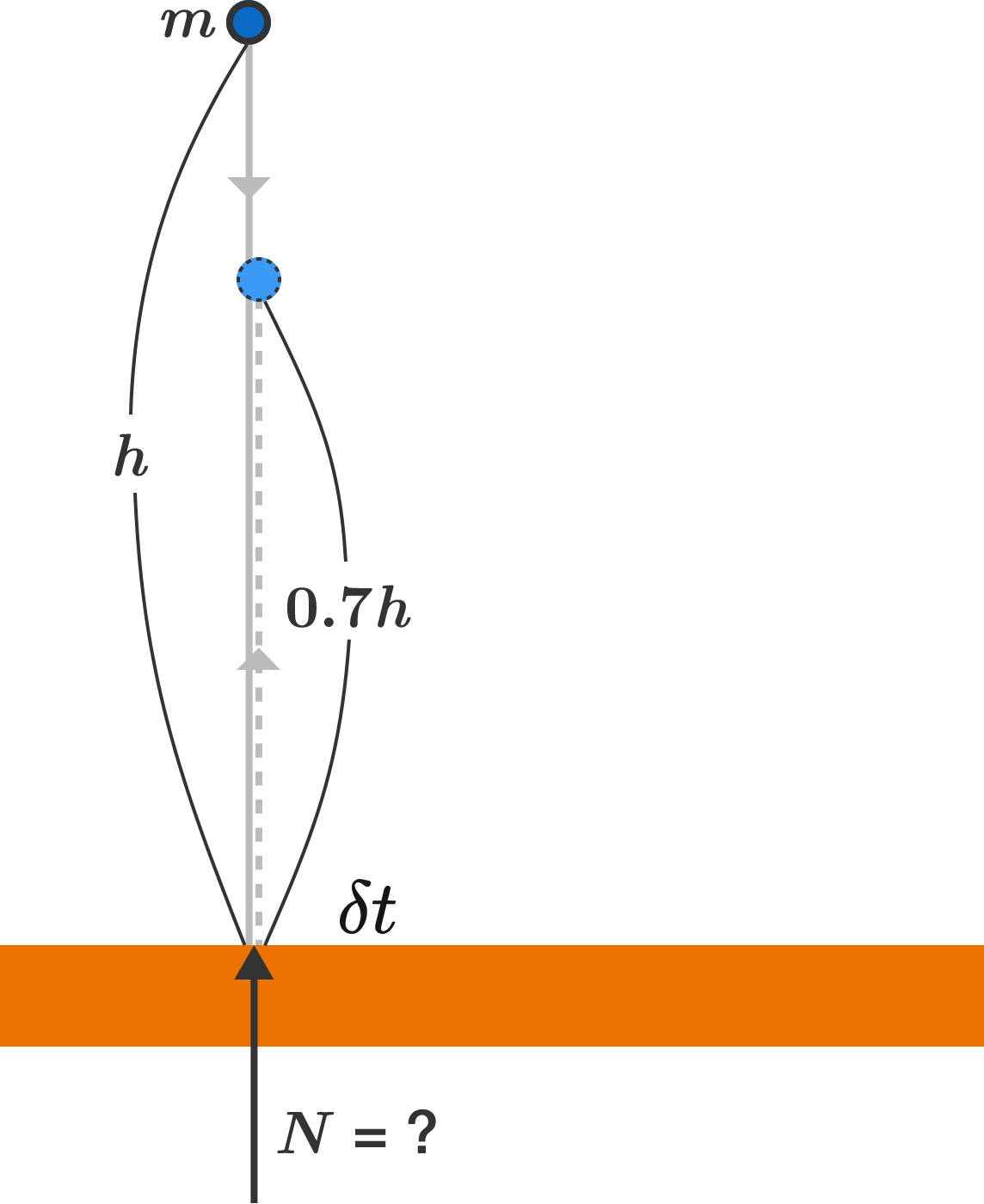# Normal bounceA ball of mass $m$ is dropped from some height in a (uniform) gravitational field of $g$. It reaches the ground and bounces directly upward, to 70% of its original height. During the bounce, the ball is in contact with the ground for a small, non-zero time interval of $\delta t$.

What can be said about the normal force between the ball and ground during the interval $\delta t?$

×